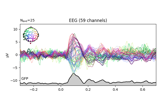# mne.channels.combine_channels¶

mne.channels.combine_channels(inst, groups, method='mean', keep_stim=False, drop_bad=False)[source]

Combine channels based on specified channel grouping.

Parameters
instinstance of `Raw`, `Epochs`, or `Evoked`

An MNE-Python object to combine the channels for. The object can be of type Raw, Epochs, or Evoked.

groups`dict`

Specifies which channels are aggregated into a single channel, with aggregation method determined by the `method` parameter. One new pseudo-channel is made per dict entry; the dict values must be lists of picks (integer indices of `ch_names`). For example:

```groups=dict(Left=[1, 2, 3, 4], Right=[5, 6, 7, 8])
```

Note that within a dict entry all channels must have the same type.

method

Which method to use to combine channels. If a `str`, must be one of ‘mean’, ‘median’, or ‘std’ (standard deviation). If callable, the callable must accept one positional input (data of shape ```(n_channels, n_times)```, or `(n_epochs, n_channels, n_times)`) and return an `array` of shape `(n_times,)`, or ```(n_epochs, n_times)```. For example with an instance of Raw or Evoked:

```method = lambda data: np.mean(data, axis=0)
```

Another example with an instance of Epochs:

```method = lambda data: np.median(data, axis=1)
```

Defaults to `'mean'`.

keep_stimbool

If `True`, include stimulus channels in the resulting object. Defaults to `False`.

If `True`, drop channels marked as bad before combining. Defaults to `False`.
combined_instinstance of `Raw`, `Epochs`, or `Evoked`
An MNE-Python object of the same type as the input `inst`, containing one virtual channel for each group in `groups` (and, if `keep_stim` is `True`, also containing stimulus channels).
## Examples using `mne.channels.combine_channels`¶